# Spinodal decomposition

A term introduced in the physical description of unmixing of metallic alloys by J.W. Cahn [a1], cf. Fig.a1.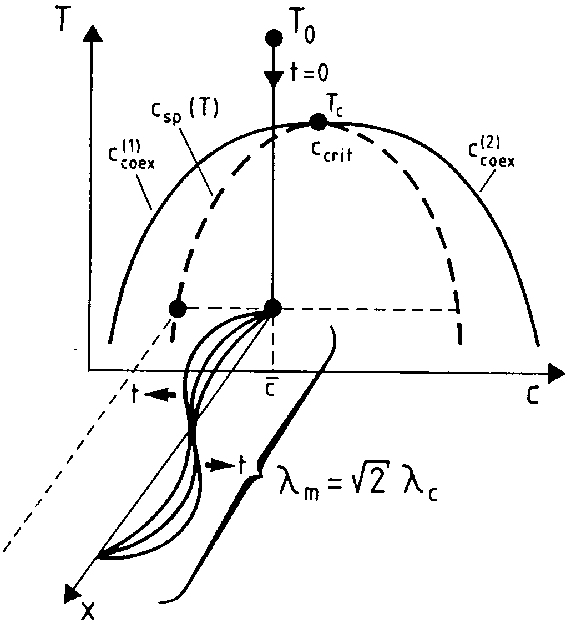Figure: s110240a

Schematic plot of a "quenching experiment" of a mixture and the resulting build-up of concentration fluctuations in a mixture. Shown is the temperature () versus concentration () plane, while the third axis () is a spacial coordinate.

The thermodynamic state of such a mixture is described by the variables temperatureand relative concentration, and one considers a situation where in the "phase diagram" of this system one finds a "miscibility gap" , i.e. there is a curve in the-plane in Fig.a1 (this curve is labelled by the two branches,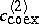in Fig.a1, which merge in a critical point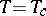,) underneath which the system cannot exist in a state of homogeneous concentrationin thermal equilibrium, while it does exist in such a state above this curve (e.g., in the initial state at a temperature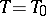,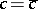in Fig.a1).

One now considers a rapid cooling experiment (quenching) where the system is brought at time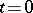from this temperatureabove the coexistence curve to a temperaturebelow the so-called spinodal curve, defined by the condition that the second derivative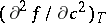of the free energy density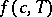vanishes. In this regime, the homogeneous state is intrinsically unstable, as is found from a linear stability analysis of concentration fluctuations [a1]. According to such a linear stability analysis, all long wavelength concentration fluctuations with wavelengths exceeding a critical wavelength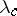are unstable, and the maximum growth rate occurs at a wavelength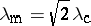. While, according to such a linear theory, one would expect that this wavelength dominates in the late stages of the phase separation process (Fig.a1), actually the process is highly non-linear [a2], and so-called "coarsening" occurs (there is a dominant wavelength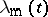, but it depends upon the timeafter the start of the process,as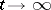[a2]).

If the thermal fluctuations are neglected, spinodal decomposition is described by the following non-linear diffusion equation for the local concentration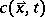at a point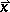of-dimensional infinitely extended space: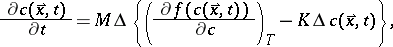(a1)

wherehas the physical meaning of a mobility,comes from a gradient energy contribution,denotes the Laplace operator, and the free energy densitycan, e.g., be written in terms of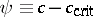aswith,constants. A parabolic spinodal curve results from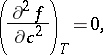The linear stability analysis of (a1) yields, writing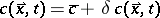,(a2)

and is solved by introducing spacial Fourier transforms,, i.e., one finds an exponential time dependence (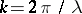),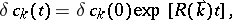(a3)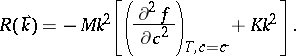One sees from (a3) that the rateis positive if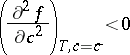and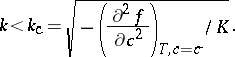The "critical wavelength" mentioned above is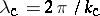.

Of course, it is clear that the linear analysis is relevant at best for the early stages of the process. Solving the full non-linear partial differential equations (a1) is a challenging problem of numerical mathematics. For applications in physics additional complications occur: in the initial stages of unmixing, the deterministic equation (a1) needs to be amended by a stochastic "random force" term to represent statistical fluctuations. In addition there occurs a coupling to further dynamical variables (in solids: the elastic displacement field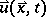; in fluid mixtures: the velocity field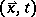). Nevertheless, (a1) is a very useful starting point, and numerous experimental applications exist [a2].